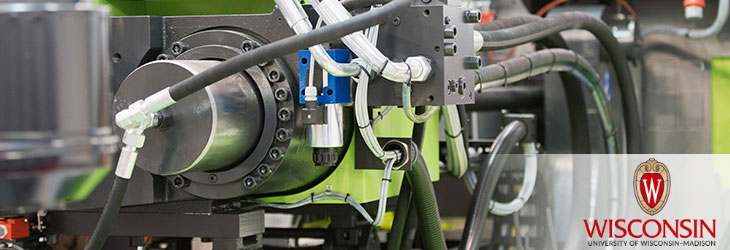Wisconsin Alumni Research Foundation

# WARF TECHNOLOGIES

EngineeringController For Power Converter
WARF: P170166US01

Inventors: Robert Lorenz, Yang Xu, Ryo Iida, Yukai Wang

The Invention
A controller includes a torque command value calculation module configured to calculate a torque command based on a speed command of the motor, an output voltage controlling module configured to control an output voltage of the power converter based on the torque command calculated by the torque command value calculation module, a voltage command value correcting module configured to correct a voltage command to the power converter based on a measured output voltage from the power converter, a flux estimation module configured to estimate stator flux and rotor flux of the motor in a subsequent control period based on the voltage command by the voltage command value correcting module and a measured current of the stator and a motor speed estimation module configured to estimate a speed of the motor in a subsequent control period based on the flux estimated by the flux estimation module.
Tech Fields